Ch 1. Limits Multimedia Engineering Math Limit of aSequence Limit of aFunction LimitLaws Continuity RateChange
 Chapter 1. Limits 2. Derivatives I 3. Derivatives II 4. Mean Value 5. Curve Sketching 6. Integrals 7. Inverse Functions 8. Integration Tech. 9. Integrate App. 10. Parametric Eqs. 11. Polar Coord. 12. Series Appendix Basic Math Units Search eBooks Dynamics Fluids Math Mechanics Statics Thermodynamics Author(s): Hengzhong Wen Chean Chin Ngo Meirong Huang Kurt Gramoll ©Kurt GramollMATHEMATICS - CASE STUDY SOLUTION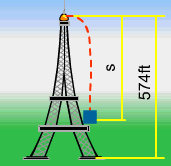Position of the Pliers The pliers fall from the top of a 574 feet tower. Its position is described by s = 16t2. What is the velocity when the pliers falls 1 second and what is the velocity when the pliers reaches the ground? First, find the velocity in terms of t seconds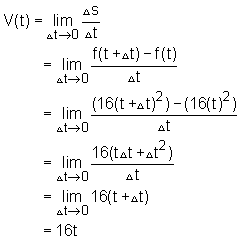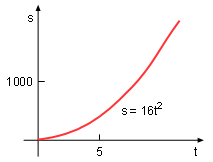Diagram of s = 16t2 At 1 seconds,      v(t = 1) = 16 feet/second Next, find the how many seconds (tg) it takes for the pliers need to reach the ground.      574 = 16t2      tg = 5.99 second Thus the velocity of pliers to reach the ground is:      v(g) = 16tg = 95.8 feet/second Discussion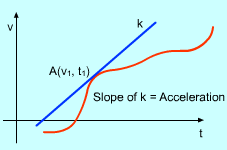Velocity and Acceleration The velocity of the pliers is calculated by the method of limit. Is it possible to calculate the acceleration? The answer is yes. Using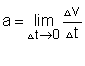In other words, the slope of the tangent line of the v-t curve at a specific point equals to the acceleration at that point.